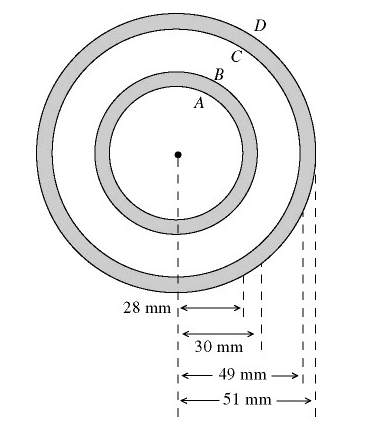# Two conducting cables - Gauss' Law

kbwelch17

## Homework Statement

The cross section of a long coaxial cable is shown in the figure, with radii as given. The linear charge density on the inner conductor is -80 nC/m and the linear charge density on the outer conductor is -10 nC/m. The inner and outer cylindrical surfaces are respectively denoted by A, B, C, and D, as shown. (ε 0 = 8.85 × 10-12 C2/N · m2) What is the magnitude of the electric field at a point that is 61 mm from the axis?## Homework Equations

Electric Flux = ∫E dot dA = Qencl/ε 0

## The Attempt at a Solution

Honestly I have no clue how to start this problem. From the problem, it looks like you need to use Gauss' Law to calculate the electric field, but in order to use Gauss' Law, don't you need electric field lines that penetrate the Gaussian surface with symmetry? These appear to be circular cylinders and would hit, a sphere for example, at different directions across the surface. The magnitude would be different at different points of the sphere, making for a difficult integral. If I were to start, then I suppose you would need to convert the charge density into total charge. In this case, the charge density of the inner conductor is -80nC/m. Since charge density is charge/area, then the total charge would be -80nC * surface area of the inner conductor which would be 2∏(1mm) * 2∏(28mm)?

Staff Emeritus
Homework Helper

## Homework Statement

The cross section of a long coaxial cable is shown in the figure, with radii as given. The linear charge density on the inner conductor is -80 nC/m and the linear charge density on the outer conductor is -10 nC/m. The inner and outer cylindrical surfaces are respectively denoted by A, B, C, and D, as shown. (ε 0 = 8.85 × 10-12 C2/N · m2) What is the magnitude of the electric field at a point that is 61 mm from the axis?## Homework Equations

Electric Flux = ∫E dot dA = Qencl/ε 0

## The Attempt at a Solution

Honestly I have no clue how to start this problem. From the problem, it looks like you need to use Gauss' Law to calculate the electric field, but in order to use Gauss' Law, don't you need electric field lines that penetrate the Gaussian surface with symmetry? These appear to be circular cylinders and would hit, a sphere for example, at different directions across the surface. The magnitude would be different at different points of the sphere, making for a difficult integral. If I were to start, then I suppose you would need to convert the charge density into total charge. In this case, the charge density of the inner conductor is -80nC/m. Since charge density is charge/area, then the total charge would be -80nC * surface area of the inner conductor which would be 2∏(1mm) * 2∏(28mm)?
Spherical symmetry isn't the only type of symmetry. You have cylindrical symmetry in this case. If you were to rotate the system around the axis, nothing really changes, right?

kbwelch17
Ah, so the Gaussian surface you would use around each is a circular cylinder?

Staff Emeritus